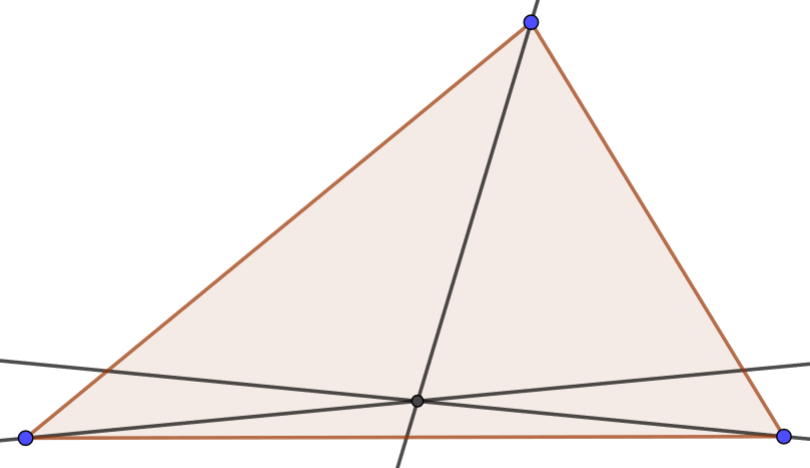# 3.3: Concurrent

$$\newcommand{\vecs}{\overset { \rightharpoonup} {\mathbf{#1}} }$$ $$\newcommand{\vecd}{\overset{-\!-\!\rightharpoonup}{\vphantom{a}\smash {#1}}}$$$$\newcommand{\id}{\mathrm{id}}$$ $$\newcommand{\Span}{\mathrm{span}}$$ $$\newcommand{\kernel}{\mathrm{null}\,}$$ $$\newcommand{\range}{\mathrm{range}\,}$$ $$\newcommand{\RealPart}{\mathrm{Re}}$$ $$\newcommand{\ImaginaryPart}{\mathrm{Im}}$$ $$\newcommand{\Argument}{\mathrm{Arg}}$$ $$\newcommand{\norm}{\| #1 \|}$$ $$\newcommand{\inner}{\langle #1, #2 \rangle}$$ $$\newcommand{\Span}{\mathrm{span}}$$ $$\newcommand{\id}{\mathrm{id}}$$ $$\newcommand{\Span}{\mathrm{span}}$$ $$\newcommand{\kernel}{\mathrm{null}\,}$$ $$\newcommand{\range}{\mathrm{range}\,}$$ $$\newcommand{\RealPart}{\mathrm{Re}}$$ $$\newcommand{\ImaginaryPart}{\mathrm{Im}}$$ $$\newcommand{\Argument}{\mathrm{Arg}}$$ $$\newcommand{\norm}{\| #1 \|}$$ $$\newcommand{\inner}{\langle #1, #2 \rangle}$$ $$\newcommand{\Span}{\mathrm{span}}$$$$\newcommand{\AA}{\unicode[.8,0]{x212B}}$$

##### Definition: Median

A line is a median if and only if it connects a vertex of a triangle to the midpoint of the opposing side.

## 3.3.1Explore

Geogebra will be helpful for performing these experiments. Be as detailed as you can with your conjectures.

Use the Geogebra example in $$\PageIndex{1}$$ to experiment with the relationship of the three perpendicular bisectors of a triangle. Move the vertices of the triangle around. What remains true about the perpendicular bisectors?Figure $$\PageIndex{1}$$: GeoGebra: Perpendicular Bisectors

Use the Geogebra example in $$\PageIndex{2}$$ to experiment with the relationship of the three medians of a triangle. Move the vertices of the triangle around. What remains true about the medians?Figure $$\PageIndex{2}$$: GeoGebra: Medians

Use the Geogebra example in $$\PageIndex{3}$$ to experiment with the relationship of the three angle bisectors of a triangle. Move the vertices of the triangle around. What remains true about the angle bisectors?Figure $$\PageIndex{3}$$: GeoGebra: Angle Bisectors

Use the Geogebra example in $$\PageIndex{4}$$ to experiment with the relationship of the three altitudes of a triangle. Move the vertices of the triangle around. What remains true about the altitudes?Figure $$\PageIndex{4}$$: GeoGebra: Altitudes

Construct △ABC. Construct △XYZ such that X-B-Y, Y-C-Z, Z-A-X and XY ll AC, YZ ll AB, ZX ll BC. Construct the perpendicular bisectors of △XYZ. What appears to be true of these with respect to △ABC.

## 3.3.2Prove

##### Lemma

Consider three points A, B, C with ℓ1 and ℓ2 the perpendicular bisectors of AB and BC respectively. Let M2 = ℓ2 ∩ BC. Show ℓ1 ll ℓ2 implies the existence of D = ℓ2 ∩ AB such that A, B and D are collinear and ∠BDM2 is a right angle.

##### Theorem: Perpendicular bisectors

Prove the conjecture about the perpendicular bisectors.

##### Theorem: Circumcenter

Three points uniquely determine a circle.

##### Lemma

Two medians intersect at a point 2/3 of the way down both medians.

##### Theorem: Medians

Prove the conjecture about the medians.

##### Theorem

A point is on the angle bisector of an angle if and only if it is equidistant from both sides of the angle.

##### Theorem: Angle bisectors

Prove the conjecture about the angle bisectors.

##### Theorem：Incenter

For each triangle there exists a circle inside and tangent to all three sides.

##### Theorem： Altitudes

Prove the conjecture about the altitudes.

This page titled 3.3: Concurrent is shared under a GNU Free Documentation License 1.3 license and was authored, remixed, and/or curated by Mark A. Fitch via source content that was edited to the style and standards of the LibreTexts platform; a detailed edit history is available upon request.